Dot Blot Analysis

There are two built in methods for analyzing a dot blot in ImageJ. The first is to treat each row as a horizontal "lane" and use ImageJ's gel analysis function. The second is to subtract the background and measure the integrated density of each dot.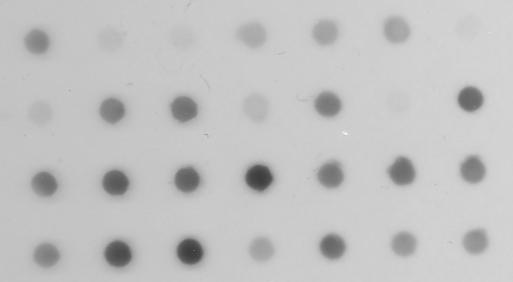Figure 1. Original dot blot.

This dot blot image is available in the File/Open Samples menu in ImageJ 1.33s or later.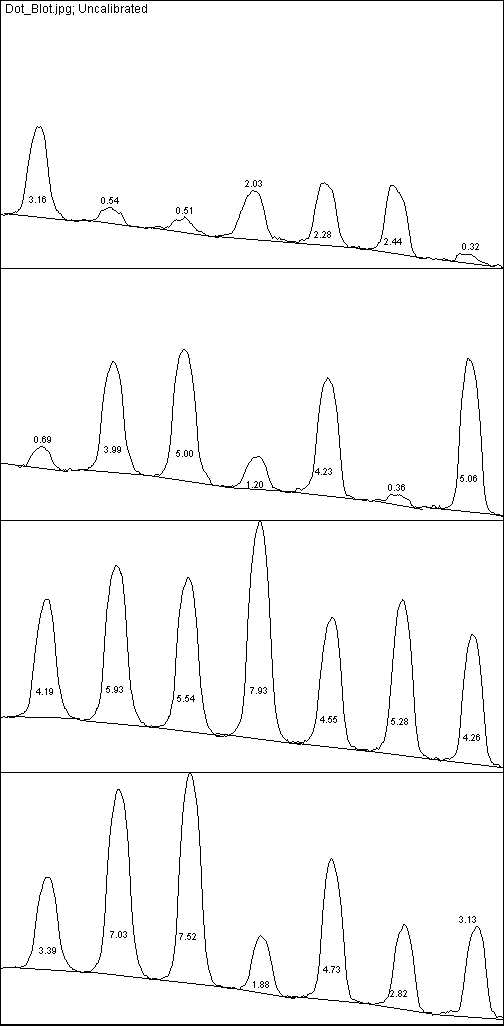Figure 2. Profile plots.

This is what you get when you treat each row in the dot blot as a horizontal "lane" and use the gel analysis procedure in the ImageJ manual. The numbers on each peak are the size of the corresponding dot as a percentage of the total size of all the dots. "Invert Peaks" in the Analyze/Gels/Gel Analyzer Options dialog was enabled to avoid having upside down peaks.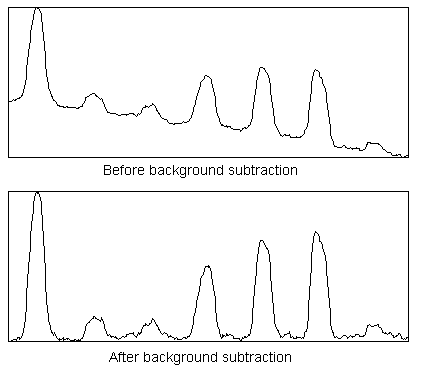Figure 3. Profile plots of first row.

In the second method, you measure the integrated density of each dot by outlining it and using the Analyze/Measure command. This method usually requires background correction of the image, which can be done using the Process/Subtract Background command. Figure 3 contains profile plots (Analyze/Plot Profile) of the first row of dots before and after background correction was done using the Subtract Background command with the rolling ball radius set to 25 pixels.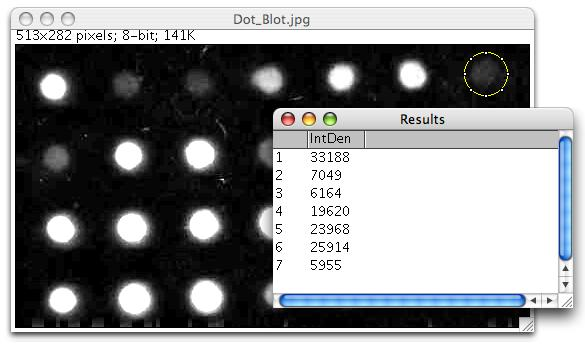Figure 4. Circular selection and integrated density measurements.

After correcting the background, enable "Integrated Density" in Analyze/Set Measurements, create a circular selection, drag it over the first dot, press "m" (Analyze/Measure), then repeat for the other 27 dots. Notice how the image now has a black background? It was inverted (Edit/Invert) so background pixel values are near zero, which is required for correct calculation of integrated density. You can invert the lookup table (Image/Lookup Tables/Invert LUT) to restore the original appearance of the image. The "Use Inverting Lookup Table" option in Edit/Options/Image will invert the pixel data and invert the lookup table.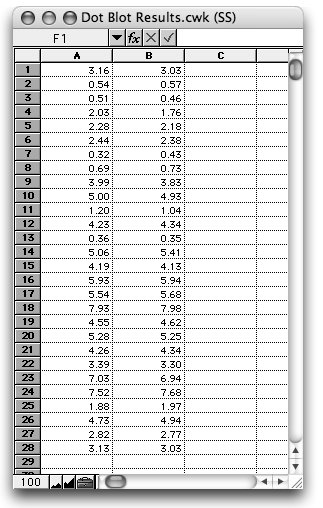Figure 5. Results using plots (A) and integrated density (B).

The first column in this spreadsheet contains the integrated densities (as a percentage of the total) of the 28 dots measured using the gel analysis procedure at rsb.info.nih.gov/ij/docs/menus/analyze.html#gels. The second contains the results obtained by doing background correction using Process/Subtract Background, selecting "Integrated Density" in Analyze/Set Measurements, and then measuring each of the 28 dots using a circular selection.

You can also analyze dot blots and microarrays using Bob Dougherty's MicroArray Profile plugin, as well as Gilles Carpentier's Dot Blot Analyzer for ImageJ toolset, available on the ImageJ website in the macros/toolsets folder and documented at image.bio.methods.free.fr/dotblot.html

| Examples | Home |